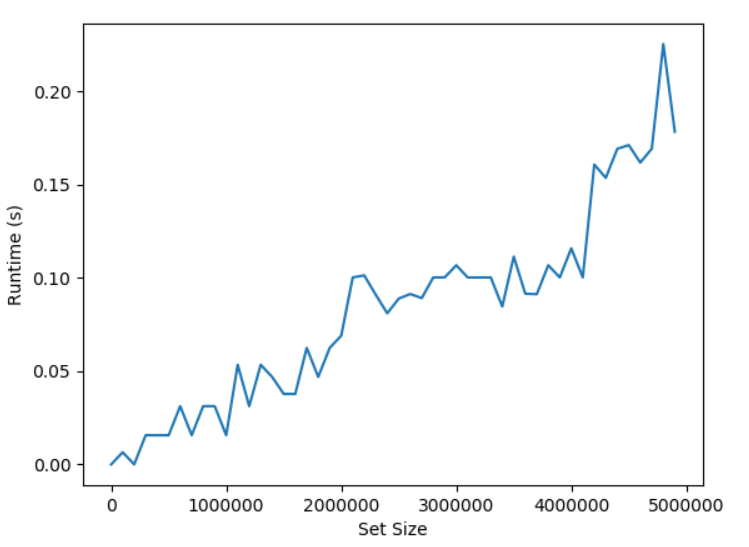# Python Set copy()

2/5 - (1 vote)

Python’s `set.copy()` method creates and returns a flat copy of this set.

Here’s a minimal example where you copy a set with two integers and a string value:

```>>> s = {1, 2, 'Alice'}
>>> s.copy()
{1, 2, 'Alice'}```

## Syntax

Let’s dive into the formal syntax of the `set.copy()` method.

`set.copy()`

The `set.copy()` method doesn’t take any argument. If you try to pass an argument, Python will raise a `TypeError: copy() takes no arguments (1 given)`. You can fix this error by not passing an argument into the method.

```>>> {1, 2, 3}.copy({'Alice', 'Bob'})
Traceback (most recent call last):
File "<pyshell#3>", line 1, in <module>
{1, 2, 3}.copy({'Alice', 'Bob'})
TypeError: copy() takes no arguments (1 given)```

## Return Value of Set copy()

The return value of `set.copy()` is a new `set` object that contains a reference to all set elements in the original set.

## Shallow vs Deep Set copy()

The `set.add()` copy operation performs a shallow copy of the set which means that each element in the set is not copied itself. The new set holds the same elements as the original set—it’s just a different container.

This has led some people to ask: how to perform a deep copy of a set?

? However, the concept of a deep copy doesn’t make any sense for sets because sets are only allowed to hold immutable objects that cannot be changed after creation. There’s no practical benefit of copying an immutable object—but tangible practical disadvantages such as memory wastage. Due to the read-only access patterns of immutable objects, the program would behave exactly the same when working on a copy compared to working on the original object. That’s why you shouldn’t even copy immutable objects but reuse them.

The following code shows that the `set.copy()` function creates a shallow copy. As checked using the “is” keyword, the copied set points to another memory location but the only set element, a tuple, points to the same memory location in both sets.

```>>> s1 = {(1, 2, 3)}
>>> s2 = s1.copy()
>>> s1.pop() is s2.pop()
True```

## What is the Time Complexity of Set copy()?

The runtime complexity of the `set.copy()` function on a set with n elements is O(n) because Python creates a new empty set and adds one element at a time to the set. This procedure is repeated n times. Each add operation is O(1) so the total runtime complexity is O(1) * O(n) which yields a linear runtime complexity.

You can see this in the following simple experiment where we run the set method multiple times for an increasing number of set elements.Figure: The runtime complexity of set.copy() grows approximately in a linear relationship with the number of elements in the set.

I ran this experiment on my Acer Aspire 5 notebook (I know) with Intel Core i7 (8th Gen) processor and 16GB of memory. Here’s the code of the experiment:

```import matplotlib.pyplot as plt
import random
import time

sizes = [i * 10**5 for i in range(50)]
runtimes = []

for size in sizes:
s = set(range(size))

# Start track time ...
t1 = time.time()
s.copy()
t2 = time.time()
# ... end track time

runtimes.append(t2-t1)

plt.plot(sizes, runtimes)
plt.ylabel('Runtime (s)')
plt.xlabel('Set Size')

plt.show()
```

## Other Python Set Methods

All set methods are called on a given set. For example, if you created a set `s = {1, 2, 3}`, you’d call `s.clear()` to remove all elements of the set. We use the term “this set” to refer to the set on which the method is executed.